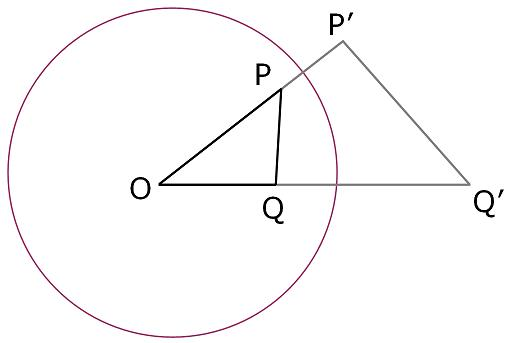#### You may also like### Fitting In

The largest square which fits into a circle is ABCD and EFGH is a square with G and H on the line CD and E and F on the circumference of the circle. Show that AB = 5EF. Similarly the largest equilateral triangle which fits into a circle is LMN and PQR is an equilateral triangle with P and Q on the line LM and R on the circumference of the circle. Show that LM = 3PQ### Look Before You Leap

Can you spot a cunning way to work out the missing length?### Triangle Midpoints

You are only given the three midpoints of the sides of a triangle. How can you construct the original triangle?

# Points in Pairs

##### Age 14 to 16Challenge Level

Two points, one inside a circle and the other outside, are related in the following way :

A line starting at the centre of the circle and passing through the first point ($P$) goes on to pass through the second point ($P'$)

Positions along the line are such that the ratio of $OP$ to the radius of the circle matches the ratio of the radius of the circle to $OP'$

For example if $OP$ happened to be $2/3$ of the radius then $OP'$ would be $3/2$ of the radius.

You can use the interactivity below to help you explore how the positions of a pair of points relate to each other.

Once you have a feel for how the points $P$ and $P'$ relate to each other, use the checkbox to add a point $Q$ and its corresponding point $Q'$.

What do you notice about triangles $OPQ$ and $OQ'P'$, where $O$ is the origin?

Final challenge
In this diagram, the radius of the circle is 10 units, $OP$ is 8 units and $OQ$ is 6 units.If the distance $PQ$ is 5 units what is the distance $P'Q'$?